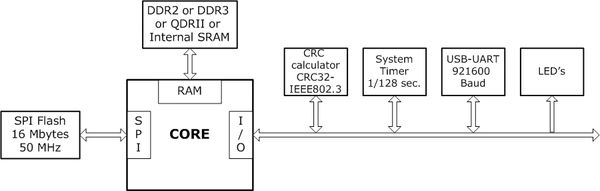#### Single core system configuration

The basic configuration of a single core system consists of:

2. Main memory system. DDR2, DDR3, QDRII or internal 512 KByte SRAM
3. 16 MByte of SPI Flash. It used as file storage.
4. Hardwired CRC-calculator used for CRC calculation according to the IEEE802.3 standard.
5. The system timer generates system ticks 128 times per second, and also contains a register-counter of these ticks.
6. 921600 Baud UART and USB to UART FT232 converter.
7. LED's for status indication.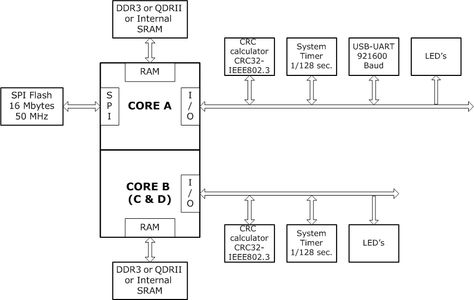#### Multi-core system configuration

In multi-core configurations, only core A has a SPI interface and a UART controller.

## Arria II board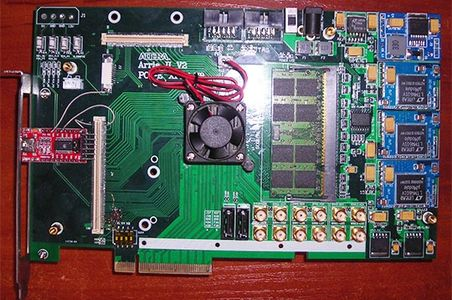Device EP2AGX125EF29I3

1GB DDR2 SDRAM.

Only the CoreOneV0 core was loaded onto this board. The core was supplemented with a DDR2 400 SDRAM (Altera ALTMEMPHY core) controller,

Logic utilization  = 96 %
Dedicated logic registers = 42839 / 99280 ( 43 % )
Total block memory bits = 2759078 / 6727680 ( 41 % )
DSP block 18-bit elements = 92 / 576 ( 16 % )

Slow timing model = 110 MHz
Fast timing model = 205 MHz

## Arria V board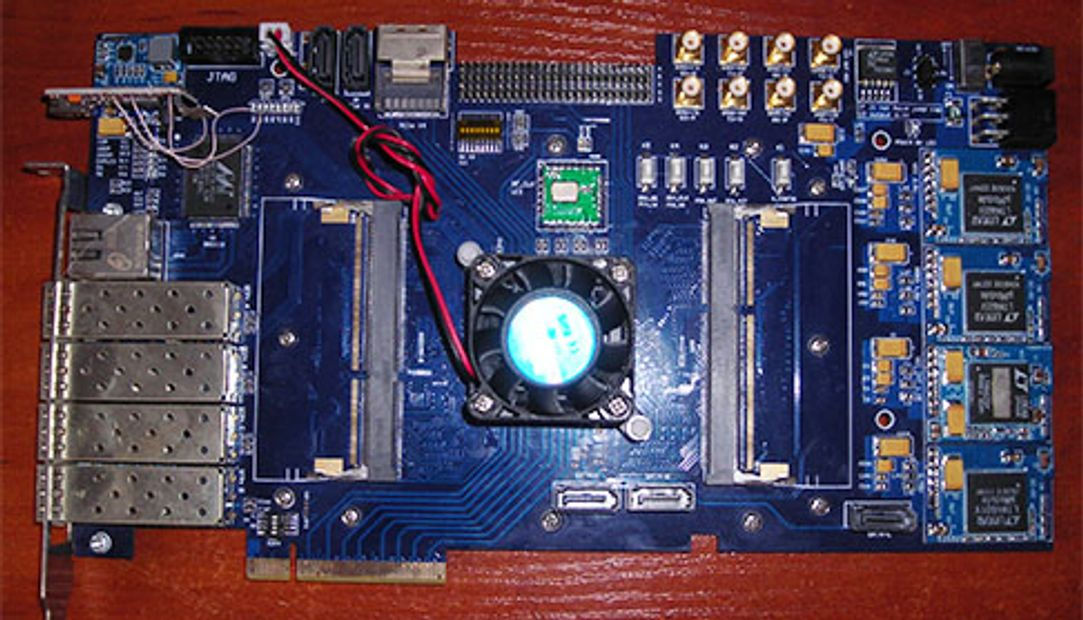Device 5AGTFC7H3F35I3.

The board has no external memory. Single-core models were equipped with 512 KB of internal FPGA-based memory. The dual-core model has 256 KB for each core.

CoreOneV0

Logic utilization (in ALMs)  = 46090 / 91680 ( 50% )
Total registers = 40298
Total block memory bits = 6303878 / 13987840 ( 45 % )
Total DSP Blocks = 41 / 800 ( 5 % )

Slow 1150mV 100C Model = 115 MHz
Slow 1150mV -40C Model = 125 MHz
Fast 1150mV 100C Model = 146 MHz
Fast 1150mV -40C Model = 189 MHz

CoreOneV2

Logic utilization (in ALMs)  = 58840 / 91680 ( 64% )
Total registers = 46221
Total block memory bits = 6304185 / 13987840 ( 45 % )
Total DSP Blocks = 77 / 800 ( 10 % )

Slow 1150mV 100C Model = 105 MHz

Slow 1150mV -40C Model = 122 MHz

Fast 1150mV 100C Model = 130 MHz

Fast 1150mV -40C Model = 167 MHz

Logic utilization (in ALMs)  = 68571 / 91680 ( 75% )
Total registers = 50572
Total block memory bits = 6714103 / 13987840 ( 48 % )
Total DSP Blocks = 41 / 800 ( 5 % )

Slow 1150mV 100C Model = 100 MHz

Slow 1150mV -40C Model = 113 MHz

Fast 1150mV 100C Model = 123 MHz

Fast 1150mV -40C Model = 158 MHz

CoreOneV0 X32 architecture

Logic utilization (in ALMs)  = 63417 / 91680 ( 69% )
Total registers = 43861
Total block memory bits = 6817048 / 13987840 ( 49 % )
Total DSP Blocks = 53 / 800 ( 7 % )

Slow 1150mV 100C Model = 94 MHz

Slow 1150mV -40C Model = 106 MHz

Fast 1150mV 100C Model = 117 MHz

Fast 1150mV -40C Model = 152 MHz

CoreOneV0DualFFT

Logic utilization (in ALMs)  = 47502 / 91680 ( 52% )
Total registers = 43464
Total block memory bits = 6310662 / 13987840 ( 45 % )
Total DSP Blocks = 45 / 800 ( 6 % )

Slow 1150mV 100C Model = 109 MHz

Slow 1150mV -40C Model = 120 MHz

Fast 1150mV 100C Model = 136 MHz

Fast 1150mV -40C Model = 174 MHz

CoreDuoV0

Logic utilization (in ALMs)  = 85560 / 91680 ( 93% )
Total registers = 74726
Total block memory bits = 8379452 / 13987840 ( 60 % )
Total DSP Blocks = 82 / 800 ( 10 % )

Slow 1150mV 100C Model = 102 MHz

Slow 1150mV -40C Model = 116 MHz

Fast 1150mV 100C Model = 126 MHz

Fast 1150mV -40C Model = 161 MHz

## Stratix V DE5-Net board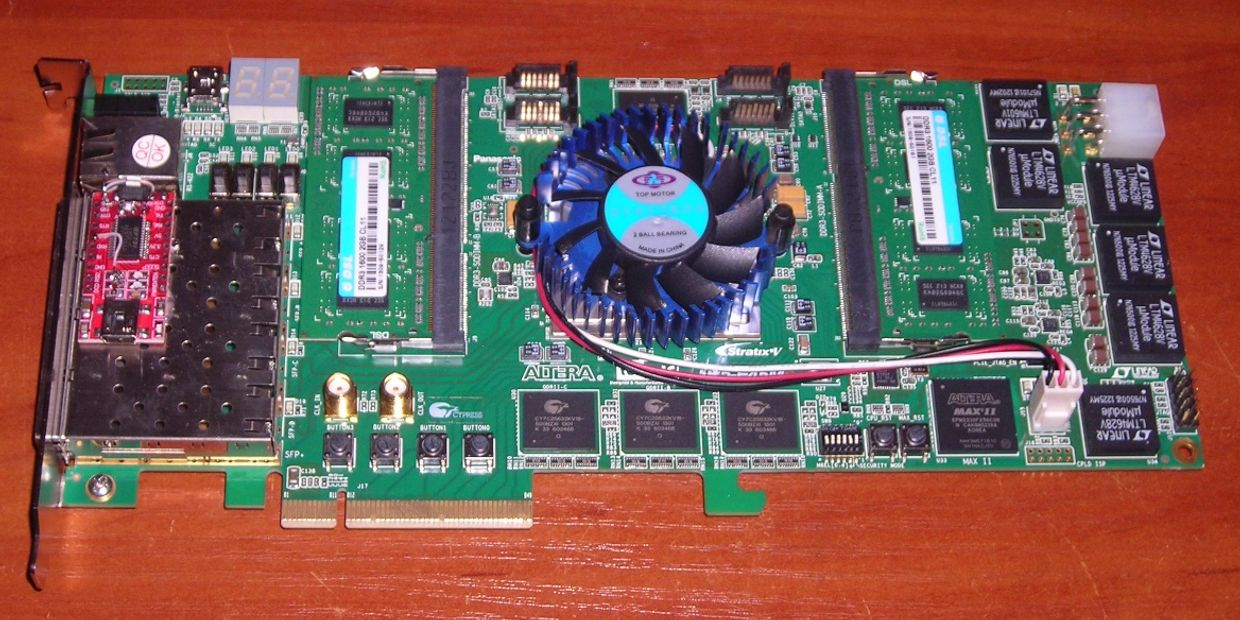Device 5SGXEA7N2F45C2

Two banks of DDR3 memory 2 GB in each slot. Four 8Mbyte QDRII chips. Intel IP functions of the DDR3 and QDRII memory controllers with the Avalon interface were used to connect the processor cores to the corresponding types of memory.

CoreOneV0

QDR Memory

Logic utilization (in ALMs)  = 46616 / 234720 ( 20 % )

Total registers = 41342

Total block memory bits = 2084560 / 52428800 ( 4 % )

Total DSP Blocks =41 / 256 ( 16 % )

Slow 900mV 85C Model = 176 MHz

Slow 900mV 0C Model = 188 MHz

Fast 900mV 85C Model = 255 MHz

Fast 900mV 0C Model = 279 MHz

DDR3 Memory

Logic utilization (in ALMs)  = 50665 / 234720 ( 22 % )

Total registers = 48634

Total block memory bits = 2944720 / 52428800 ( 6 % )

Total DSP Blocks =41 / 256 ( 16 % )

Slow 900mV 85C Model = 164 MHz

Slow 900mV 0C Model = 174 MHz

Fast 900mV 85C Model = 243 MHz

Fast 900mV 0C Model = 265 MHz

CoreOneV0 X32

DDR3 Memory

Logic utilization (in ALMs)  = 73828 / 234720 ( 31 % )

Total registers = 54828

Total block memory bits = 3483536 / 52428800 ( 7 % )

Total DSP Blocks =49 / 256 ( 19 % )

Slow 900mV 85C Model = 156 MHz

Slow 900mV 0C Model = 166 MHz

Fast 900mV 85C Model = 227 MHz

Fast 900mV 0C Model = 247 MHz

Internal 512KB SSRAM mode

Logic utilization (in ALMs)  = 64214 / 234720 ( 27 % )

Total registers = 45337

Total block memory bits = 6817680 / 52428800 ( 13 % )

Total DSP Blocks =49 / 256 ( 19 % )

Slow 900mV 85C Model = 158 MHz

Slow 900mV 0C Model = 170 MHz

Fast 900mV 85C Model = 229 MHz

Fast 900mV 0C Model = 244 MHz

QDRII Memory 8Mb per core

Logic utilization (in ALMs)  = 180244 / 234720 ( 77 % )

Total registers = 157462

Total block memory bits = 12617456 / 52428800 ( 24 % )

Total DSP Blocks =164 / 256 ( 64 % )

Slow 900mV 85C Model = 164 MHz

Slow 900mV 0C Model = 176 MHz

Fast 900mV 85C Model = 238 MHz

Fast 900mV 0C Model = 261 MHz

DDR3 Memory

Logic utilization (in ALMs)  = 206629 / 234720 ( 88 % )

Total registers = 133787

Total block memory bits = 6437980 / 52428800 ( 12 % )

Total DSP Blocks =180 / 256 ( 70 % )

Slow 900mV 85C Model = 150 MHz

Slow 900mV 0C Model = 163 MHz

Fast 900mV 85C Model = 216 MHz

Fast 900mV 0C Model = 234 MHz#### Kintex 7 Board

Device XC7K420

Implemented CoreOneV0 runs at 100 MHz.

Internal SRAM 512 Kb

FPGA resources:

LUT - 62572 / 260600 (24%)

LUTRAM -  5091 / 108600 (4.69%)

FF - 29705 / 521200 (5.70%)

BRAM - 179.5 / 835 (21.5%)

DSP - 46 / 1680 (2.74)